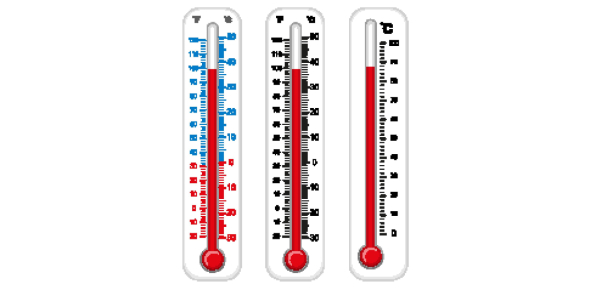# Chemistry Heat And Temperature Quiz

10 Questions | Attempts: 3909
ShareSettingsGet ready for this amazing Chemistry Heat and Temperature Quiz. It takes into consideration the resultant heat and temperatures of different reactions and elements. How good are you with the topic? Take the quiz and find out. If you manage to get a score that is equal to or above 70, then you can consider yourself passed in this quiz with a good score. Let us see what score you get!

• 1.
How many joules of heat is required to heat 68.00 grams of aluminum foil from 55.00 °C to 93.00 °C in case aluminum has a specific heat of 0.90 J/g °C?
• A.

2326 J

• B.

2871 J

• C.

3420 J

• D.

9058 J

• 2.
What is the final temperature of a   91.0 g piece of copper, with an initial temperature of 20.0 °C, after absorbing 1581 J of heat?  Copper has a specific heat of 0.386 J/g °C.
• A.

65.0 °C

• B.

25.0°C

• C.

45.0°C

• D.

703 °C

• 3.
What is the specific heat of gold if a 38.0-gram bracelet releases 460.8 Joules of heat energy and the increase in temperature is 94.0 °C?
• A.

0.129 J/g °C

• B.

7.75 J/g °C

• C.

4.18 J/g °C

• D.

186 J/g °C

• 4.
The temperature of an element affects its weight.
• A.

True

• B.

False

• 5.
202.8 J of heat is released from a piece of silver while cooling 65.0 °C.  What's the mass of the sample?  Silver has a specific heat of 0.240 J/g °C.
• A.

13.0 grams

• B.

0.748 grams

• C.

3.12 grams

• D.

4.18 grams

• 6.
250.8 J of heat is released from a piece of gold while cooling 65.0 °C. 13.0 grams is the mass of the sample. Gold has a specific heat of 0.240 J/g °C.
• A.

True

• B.

False

• 7.
3780.8 J of heat is released from a piece of gold while cooling 25.0 °C. 11.0 grams is the mass of the sample. Gold has a specific heat of 0.670 J/g °C.
• A.

True

• B.

False

• 8.
Which is the definition of calorimetry?
• A.

The science of calculating the heat of chemical reactions and physical changes

• B.

The amount of energy needed to heat 1 cm of water 1 °C

• C.

The science used to quantify and describe physically the human color perception

• D.

The amount of energy transferred from one body to another due to thermal contact

• 9.
350.8 J of heat is released from a piece of silver while cooling 55.0 °C. 11.0 grams is the mass of the sample. Silver has a specific heat of 0.6220 J/g °C.
• A.

True

• B.

False

• 10.
What is the change in temperature if a 70.0-gram sample of nickel absorbs 1756 J of energy? Nickel has a specific heat of 0.440 J/g °C.
• A.

57.0 °C

• B.

11.0 °C

• C.

25.1 °C

• D.

30.8 °C

## Related TopicsBack to top
×

Wait!
Here's an interesting quiz for you.